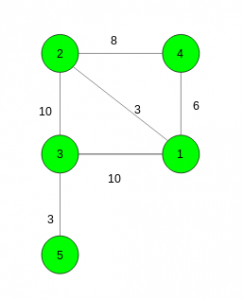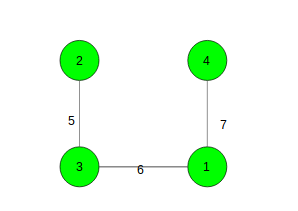# Shortest path from source to destination such that edge weights along path are alternatively increasing and decreasing

• Difficulty Level : Hard
• Last Updated : 24 Apr, 2019

Given a connected graph with N vertices and M edges. The task is to find the shortest path from source to the destination vertex such that the difference between adjacent edge weights in the shortest path change from positive to negative and vice versa ( Weight(E1) > Weight(E2) < Weight(E3) …. ). If no such path exists then print -1.

Examples:

Attention reader! Don’t stop learning now. Get hold of all the important DSA concepts with the DSA Self Paced Course at a student-friendly price and become industry ready.  To complete your preparation from learning a language to DS Algo and many more,  please refer Complete Interview Preparation Course.

In case you wish to attend live classes with experts, please refer DSA Live Classes for Working Professionals and Competitive Programming Live for Students.

Input: source = 4, destination = 3Output: 19
4 – 2 – 1 – 3 (Edge Weights: 8, 3, 10) and 4 – 1 – 2 – 3 (Edge Weights: 6, 3, 10) are the only valid paths.
Second path takes the minimum cost i.e. 19.

Input: source = 2, destination = 4Output: -1
No such path exists.

## Recommended: Please try your approach on {IDE} first, before moving on to the solution.

Approach: Here, We need to keep two copies of adjacent lists one for positive difference and other for negative difference. Take a Priority Queue as in Dijkstras Algorithm and keep four variables at a time i.e.,

1. cost: To store the cost of the path till current node.
2. stage: An integer variable to tell what element needs to be taken next, if the previous value was negative then a positive value needs to be taken else take negative.
3. weight: Weight of the last visited node.
4. vertex: Last visited vertex.

For every vertex push the adjacent vertices based on the required condition (value of stage). See the code for better understanding.

Below is the implementation of the above approach:

 `// C++ implementation of the approach ` `#include ` `using` `namespace` `std; ` `#define N 100005 ` ` `  `// To store the graph ` `vector > incr[N], decr[N]; ` `int` `_incr[N], _decr[N], shortest[N]; ` ` `  `int` `n, m, src, dest, MAXI = 1LL << 30; ` ` `  `// Function to add edges ` `void` `Add_edge(``int` `x, ``int` `y, ``int` `w) ` `{ ` `    ``incr[x].push_back({ w, y }); ` `    ``incr[y].push_back({ w, x }); ` `    ``decr[x].push_back({ -w, y }); ` `    ``decr[y].push_back({ -w, x }); ` `} ` ` `  `// Function to find the shortest distance from ` `// source to destination ` `int` `Modified_Dijkstra() ` `{ ` ` `  `    ``// Total cost, stage, weight of previous, vertex ` `    ``priority_queue, pair<``int``, ``int``> > > q; ` ` `  `    ``// Sort the edges ` `    ``for` `(``int` `i = 1; i <= n; i++) { ` `        ``sort(incr[i].begin(), incr[i].end()); ` `        ``sort(decr[i].begin(), decr[i].end()); ` `    ``} ` ` `  `    ``for` `(``int` `i = 1; i <= n; i++) ` `        ``shortest[i] = MAXI; ` ` `  `    ``// Push the source vertex ` `    ``q.push({ { 0, 0 }, { 0, src } }); ` ` `  `    ``while` `(!q.empty()) { ` ` `  `        ``// Take the top element in the queue ` `        ``pair, pair<``int``, ``int``> > FRONT = q.top(); ` ` `  `        ``// Remove it from the queue ` `        ``q.pop(); ` ` `  `        ``// Store all the values ` `        ``int` `cost = -FRONT.first.first; ` `        ``int` `stage = FRONT.first.second; ` `        ``int` `weight = FRONT.second.first; ` `        ``int` `v = FRONT.second.second; ` ` `  `        ``// Take the minimum cost for the vertex ` `        ``shortest[v] = min(shortest[v], cost); ` ` `  `        ``// If destination vertex has already been visited ` `        ``if` `(shortest[dest] != MAXI) ` `            ``break``; ` ` `  `        ``// To make difference negative ` `        ``if` `(stage) { ` ` `  `            ``// Start from last not visited vertex ` `            ``for` `(``int` `i = _incr[v]; i < incr[v].size(); i++) { ` ` `  `                ``// If we can take the ith vertex ` `                ``if` `(weight > incr[v][i].first) ` `                    ``q.push({ { -(cost + incr[v][i].first), 0 }, ` `                             ``{ incr[v][i].first, incr[v][i].second } }); ` `                ``else` `{ ` ` `  `                    ``// To keep the last not visited vertex ` `                    ``_incr[v] = i; ` `                    ``break``; ` `                ``} ` `            ``} ` `        ``} ` ` `  `        ``// To make difference positive ` `        ``else` `{ ` ` `  `            ``// Start from last not visited vertex ` `            ``for` `(``int` `i = _decr[v]; i < decr[v].size(); i++) { ` ` `  `                ``// If we can take the ith vertex ` `                ``if` `(weight < -decr[v][i].first) ` `                    ``q.push({ { -(cost - decr[v][i].first), 1 }, ` `                             ``{ -decr[v][i].first, decr[v][i].second } }); ` `                ``else` `{ ` ` `  `                    ``// To keep the last not visited vertex ` `                    ``_decr[v] = i; ` `                    ``break``; ` `                ``} ` `            ``} ` `        ``} ` `    ``} ` ` `  `    ``if` `(shortest[dest] == MAXI) ` `        ``return` `-1; ` ` `  `    ``return` `shortest[dest]; ` `} ` ` `  `// Driver code ` `int` `main() ` `{ ` `    ``n = 5, src = 4, dest = 3; ` ` `  `    ``// Adding edges ` `    ``Add_edge(4, 2, 8); ` `    ``Add_edge(1, 4, 6); ` `    ``Add_edge(2, 3, 10); ` `    ``Add_edge(3, 1, 10); ` `    ``Add_edge(1, 2, 3); ` `    ``Add_edge(3, 5, 3); ` ` `  `    ``cout << Modified_Dijkstra(); ` ` `  `    ``return` `0; ` `} `

Output:

```19
```

My Personal Notes arrow_drop_up
Recommended Articles
Page :